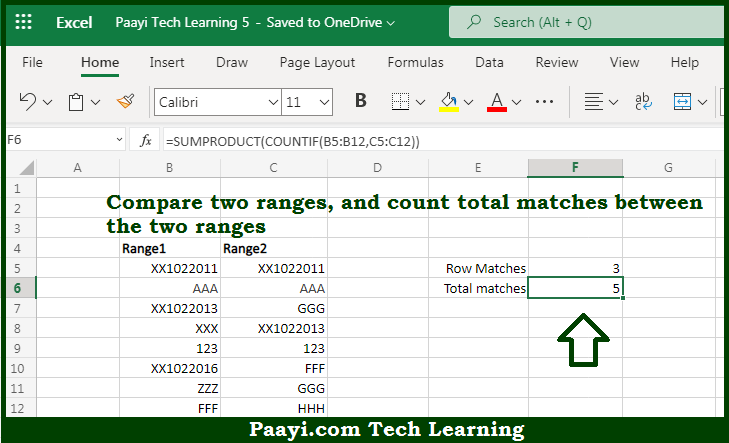# Learn How to COUNT Total Matches in Two Ranges in Microsoft Excel

Written by | 0 Comments | 584 Views

In this article, you will learn how to COUNT various things in Microsoft Excel using a single or combination of functions and its purpose. You will also get to know how to COUNT Total Matches in Two Ranges and see the generic formula.

COUNT Total Matches in Two Ranges in Microsoft Excel

The main purpose of this formula is to count total matches between the two ranges and compare the two ranges. Here we will learn how to count two matches in two ranges in Microsoft Excel. That implies, with the help of a formula based on the SUMPRODUCT and COUNTIF function you can able to count total matches between the two ranges and compare two ranges. So, with the help of this formula, you can able to count total matches between the two ranges and compare the two ranges.

General Formula to COUNT Total Matches in Two Ranges

=SUMPRODUCT(COUNTIF(range1,range2))

The Explanation for the COUNT Total Matches in Two RangesSo we know that with the help of the given formula above you can able to count total matches between the two ranges and compare the two ranges. Here we will learn how to count two matches in two ranges in Microsoft Excel. As we know that the COUNTIF function will count items in a range that meet the given criteria. Usually, you will provide a COUNTIF function a range like A1:A10, and easier criteria like ">10". The COUNTIF function will then return the count of cells in A1:A10 that are greater than 10. In the example given above, we are giving COUNTIF a range for criteria, without using any logical operators. That implies the COUNTIF function will check for equivalency.  This means it will behave as if we used the equals (=) operator. So, with the help of this formula, you can able to count total matches between the two ranges and compare the two ranges.